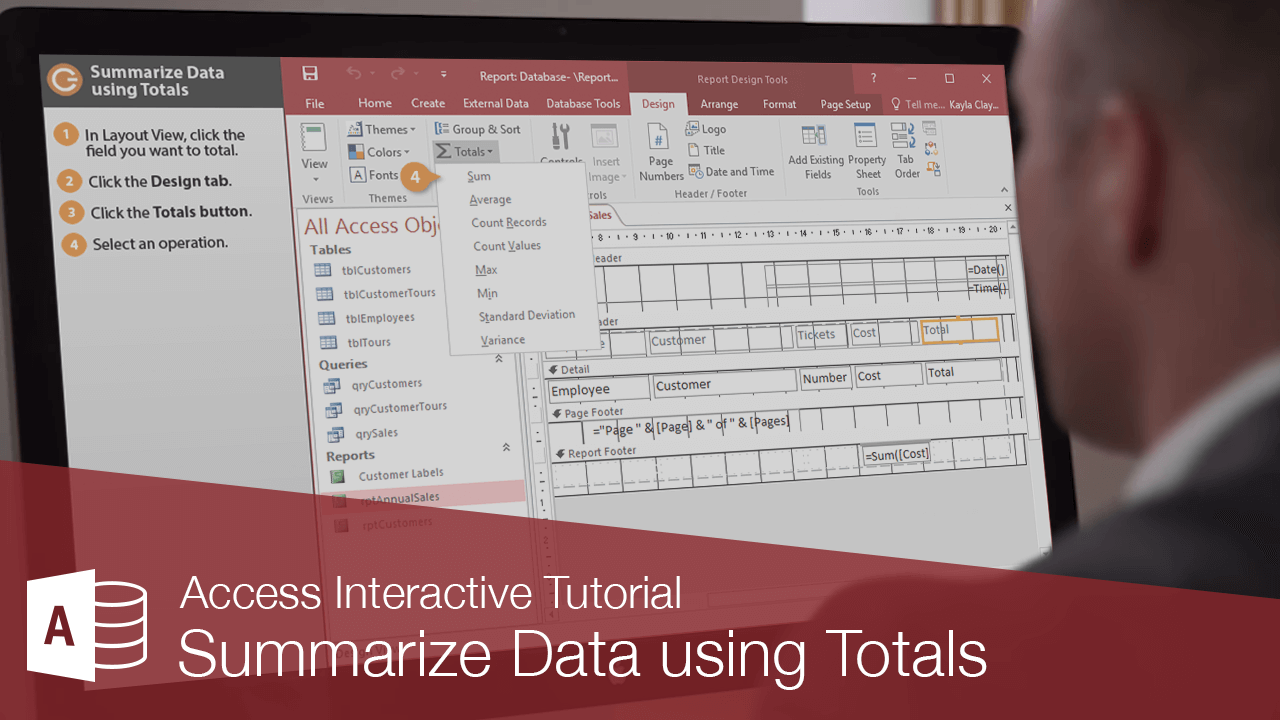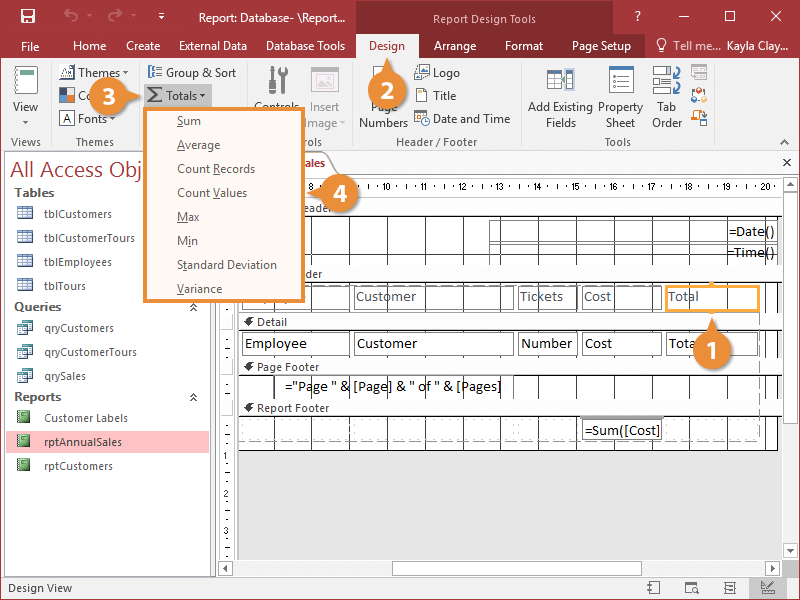Summarize Data using Totals | CustomGuide# Summarize Data using Totals

How to Summarize Data using Totals in AccessA calculated control displays totals and other arithmetic computations on a form or report. In this lesson, you'll learn how to add and use the Total feature to summarize information on a report by calculating fields to your specifications. With the Total feature you could, for example, total the number of phone calls each of your company's telemarketers made, and the number of phone calls made by the collective telemarketers at your company.

1. In Layout View or Design View, click the field you want to total.
2. Click the Design tab.
3. Click the Totals button..
4. Select an operation.

The operations that are available will vary depending on the type of data in the field. You can also modify the totals using options found on the Group, Sort, and Total pane.A grand total appears at the end of the report, and if you have created any groups in the report, group totals are added after each group as well.

Common Total Operators
Sum Totals all the values listed in a field. Sum([InvoiceTotal])
Average Calculates the average of all the values listed in a field. Avg([InvoiceTotal])
Count Records Counts the number of records. Count(*)
Count Values Counts how many values are listed in a field. Count([InvoiceTotal])
Max Finds and displays the largest value listed in a field. Max([InvoiceTotal])
Min Finds and displays the smallest value listed in a field. Min([InvoiceTotal])
Standard Deviation Finds and displays the amount of deviation from the average. StDev([InvoiceTotal])
Variance Finds and displays the variance of the data set. Var([InvoiceTotal])